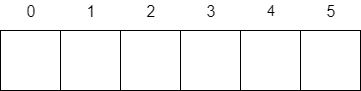#[Golang] 程式設計教學：使用陣列 (Array) 和切片 (Slice)

## 陣列 (Array)### 建立陣列

``````package main

import "log"

func main() {
var langs string

langs = "Go"
langs = "Python"
langs = "Ruby"
langs = "PHP"

if !(langs == "Go") {
log.Fatal("Wrong string")
}
}``````

``````package main

import "log"

func main() {
langs := string{
"Go",
"Python",
"Ruby",
"PHP",
}

if !(langs == "Go") {
log.Fatal("Wrong string")
}
}``````

### 走訪陣列

``````package main

import "fmt"

func main() {
langs := string{
"Go",
"Python",
"Ruby",
"PHP",
}

for i, e := range langs {
fmt.Println(fmt.Sprintf("%d: %s", i+1, e))
}
}``````

``````package main

import "fmt"

func main() {
langs := string{
"Go",
"Python",
"Ruby",
"PHP",
}

for _, e := range langs {
fmt.Println(e)
}
}``````

``````package main

import "fmt"

func main() {
arr := int{1, 2, 3, 4, 5}

for _, e := range arr {
e = e * e
}

for _, e := range arr {
fmt.Println(e)
}
}``````

``````package main

import "fmt"

func main() {
arr := int{1, 2, 3, 4, 5}

for i := 0; i < len(arr); i++ {
arr[i] = arr[i] * arr[i]
}

for _, e := range arr {
fmt.Println(e)
}
}``````

## 切片 (Slice)

### 建立切片

``````package main

import "fmt"

func main() {
langs := []string{"Go", "Python", "Ruby", "PHP"}

for _, e := range langs {
fmt.Println(e)
}
}``````

``````package main

import (
"fmt"
"log"
"reflect"
)

func main() {
langs := string{"Go", "Python", "Ruby", "PHP"}
slice := langs[0:4] // Upper bound excluded.

// Print out the types of these variables
fmt.Println(reflect.TypeOf(langs))
fmt.Println(reflect.TypeOf(slice))

if !(langs == "PHP") {
log.Fatal("Wrong value")
}

slice = "Perl"

if !(langs == "Perl") {
log.Fatal("Wrong value")
}
}``````

``````package main

import (
"log"
)

func main() {
matrix := [][]float64{
[]float64{1, 2, 3},
[]float64{4, 5, 6},
}

if !(matrix == 5) {
log.Fatal("Wrong value")
}
}``````

``````package main

import (
"fmt"
)

func main() {
slice := make([]int, 5)

for i := 0; i < len(slice); i++ {
n := i + 1
slice[i] = n * n
}

for _, e := range slice {
fmt.Println(e)
}
}``````

### 改變切片大小

``````package main

import (
"log"
)

func main() {
slice := []int{1, 2, 3, 4, 5}

if !(len(slice) == 5) {
log.Fatal("Wrong length")
}

slice = append(slice, 6, 7, 8)

if !(len(slice) == 8) {
log.Fatal("Wrong length")
}
}``````

``````package main

import (
"log"
)

func main() {
slice := []int{1, 2, 3, 4, 5}

if !(len(slice) == 5) {
log.Fatal("Wrong length")
}

if !(slice == 3) {
log.Fatal("Wrong value")
}

// Remove the 3rd element.
slice = append(slice[0:2], slice[3:5]...)

if !(len(slice) == 4) {
log.Fatal("Wrong length")
}

if !(slice == 4) {
log.Fatal("Wrong value")
}
}``````